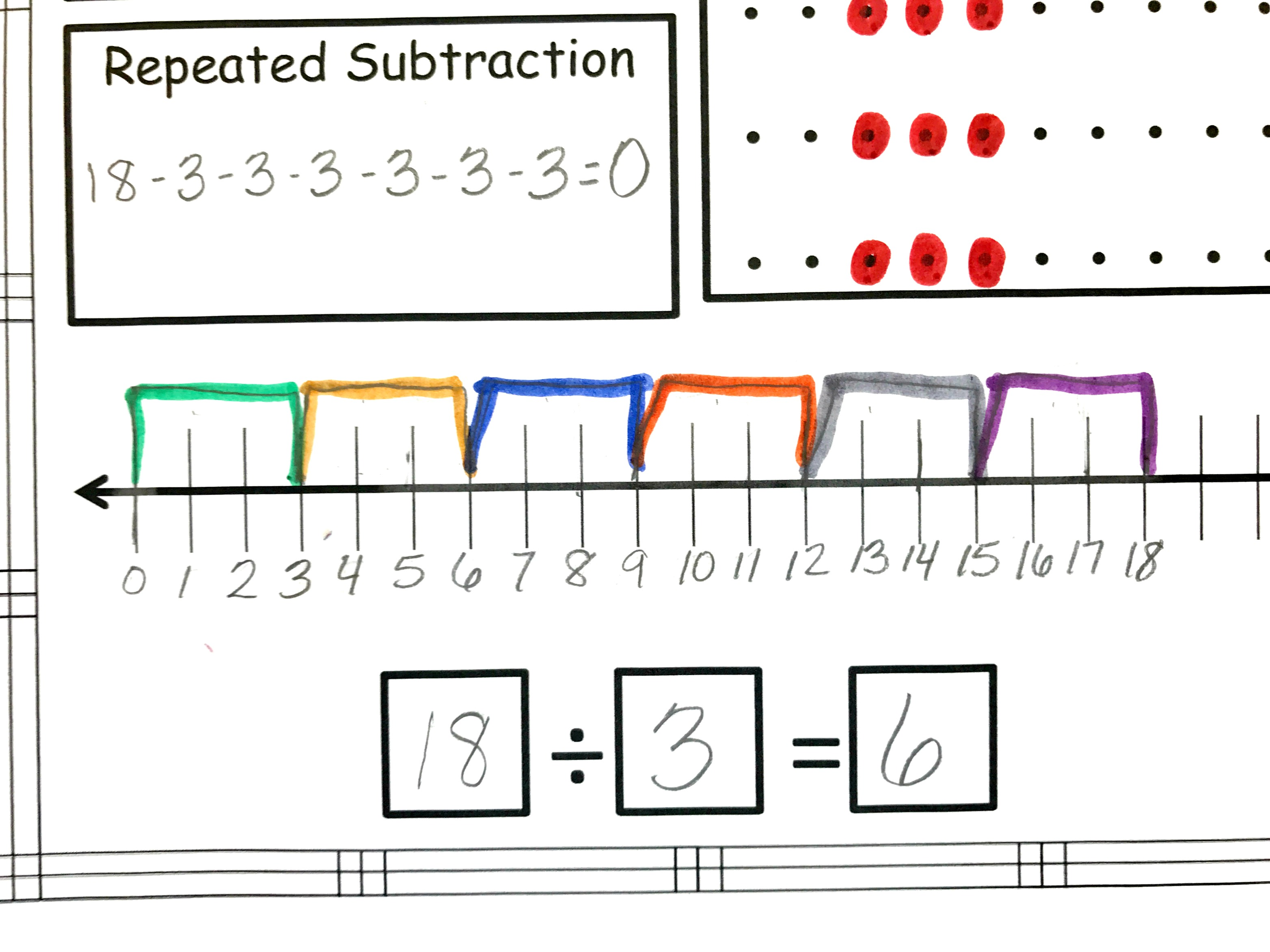Home » Math » FREE Division Word Problems with Five Strategies To Solve

# FREE Division Word Problems with Five Strategies To Solve

Are your children ready to conquer division? These interactive, division problem solving worksheets will help children solve word problems in five different ways.He has done it numerous times. Sharing a group of toys with his siblings, or even sharing a bag of M and M’s. In those situations, he instinctively knows what to do.

When we hit division problem in his math book, though, he wasn’t quite as sure what to do.

I wanted this little man of mine to be able to relate division to what he has done over and over while creating strategies for solving division problems.

These word problems with five steps were what we came up with.

*This post may contain affiliate links from Amazon and other bloggers.

### Step 1: Division Problem Solving by Grouping

The first step the way we normally teach children to solve division problems. The students grab the amounts of objects that need to be divided up and then place them in the correct amount of groups. It is very hands-on and a visual way for our children to understand what is happening when we are dividing.### Step 2: Solving Division Word Problems by Repeated Subtraction

To solve a word problem using repeated subtraction, students start with the number being divided up or the dividend. Now they subtract the divisor or the number that tells how many groups are needed from the dividend over and over until they reach zero. The number of times they subtracted is the answer.### Step 3: Division Problem Solving with Arrays

Chances are if you taught multiplication in a hands-on way, you taught is using arrays. You can create an array when you place objects, pictures, or numbers in equal columns and equal rows.

With multiplication, you would take a problem like 4 x 5, and make 4 rows with 5 in each column. You would end up with 20 objects, which of course is the answer to the multiplication problem.

Division is a little different. If the problem is 18 ÷ 3, The student creates three rows. They then keep placing one object in each row until the have used18 objects. They now have an array that is a 3 by 6. The answer to the division problem is 6.Want to know how to use arrays to divide when the numbers are larger? Check out this POST!### Step 4: Number Line

Number lines have become an important tool in helping children solve problems. The beginning of this video by Ramy Melhem clearly show how to divide using a number line, and the little frog hopping is great visual for our little ones.### Step 5: Equation

The final step is very easy after all the work above. The students simply figure out what number was divided up, and place it in the first box. They then look at how many groups the created, and that is the number that goes into the second box. Finally, they figure out how many objects were in each group and that is the answer or quotient. That number goes in the last box.

By throwing in markers and painting with q-tips these sheets were fun for my little man, and I could see his understanding of division grow.

We moved onto these cut and paste division assessments, and his thinking was challenged even more. Through all this practice he is on his way to mastering simple division problems, and your kiddos can master it too.

You’ve Got This

### Get This Cut and Paste Division Assessment at my TpT Store.Or this spin and win division printable from Life Over C’s.